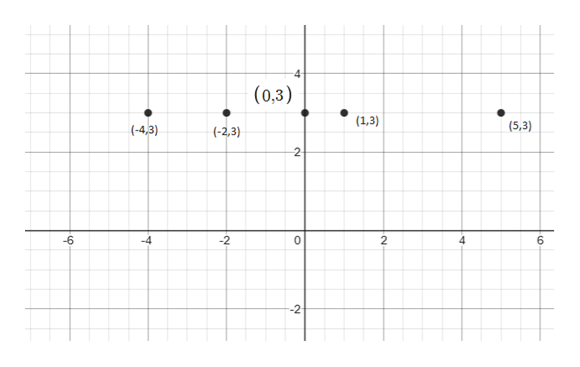I dont understant what this question is asking me to do.   plot the points (0, 3) , (1, 3) , (-2, 3) , (5, 3) , and (-4, 3). Describe the set of all points of the form (x, 3), where x is a real number.

Question

I dont understant what this question is asking me to do.

plot the points (0, 3) , (1, 3) , (-2, 3) , (5, 3) , and (-4, 3). Describe the set of all points of the form (x, 3), where x is a real number.

Step 1

Refer to the question .

First plot all the points which is also called the ordered pair where (x,y) , x represents x coordinate which is horizontal line and y is y coordinate which is vertical line the  Cartesian form of graph as,help_outlineImage Transcriptionclose4- (0,3) (1,3) (5,3) (-4,3) (-2,3) 2 -6 -4 -2 0 2 6 --2 fullscreen
Step 2

Now we have to describe the set of all points of the form (x, 3), where x is a real number.

As the real number is that numbers which cons...

Want to see the full answer?

See Solution

Want to see this answer and more?

Our solutions are written by experts, many with advanced degrees, and available 24/7

See Solution
Tagged in

Other